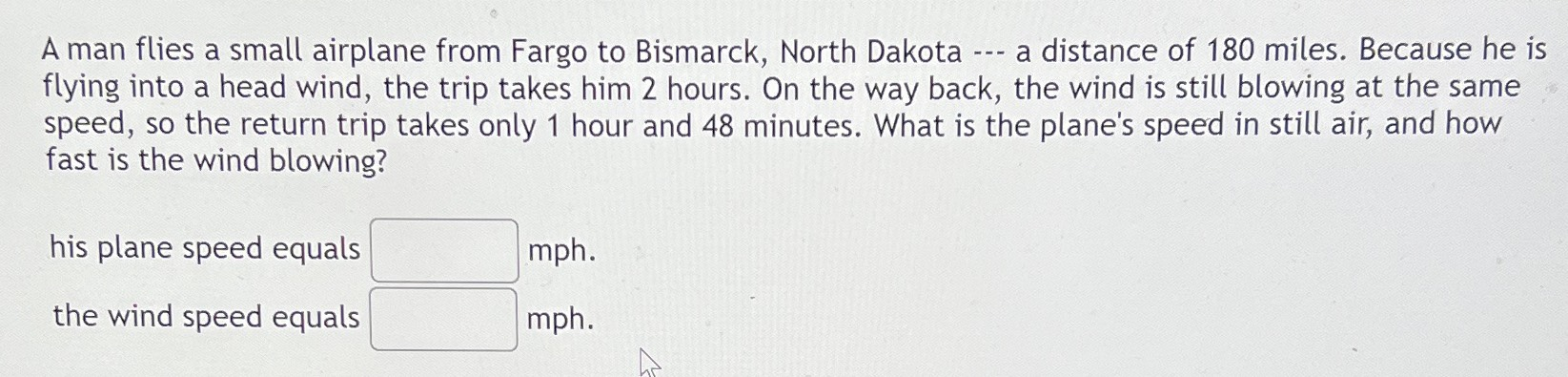### ¿Todavía tienes preguntas de matemáticas?

Pregunte a nuestros tutores expertos
Algebra
PreguntaA man flies a small airplane from Fargo to Bismarck, North Dakota... a distance of $$180$$ miles. Because he is flying into a head wind, the trip takes him $$2$$ hours. On the way back, the wind is still blowing at the same speed, so the return trip takes only $$1$$ hour and $$48$$ minutes. What is the plane's speed in still air, and how fast is the wind blowing?

his plane speed equals $$\square mph .$$

the wind speed equals $$\square mph .$$